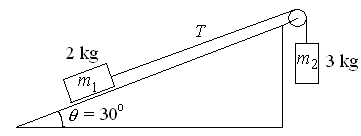First Name: ___________________ Last Name: ____________________ Section: _________

October 1, 1999 Physics 207

Exam 1

Print your name and section clearly on all five pages. (If you do not know your section number, write your TAs name.) Show all work in the space immediately below each problem. Your final answer must be placed in the box provided. Problems will be graded on reasoning and intermediate steps as well as on the final answer. Be sure to include units wherever necessary, and the direction of vectors. Each problem is worth 25 points. In doing the problems, try to be neat. Check your answers to see that they have the correct dimensions (units) and are the right order of magnitudes. You are allowed one 8½ x 11" sheet of notes and no other references. The exam lasts exactly 50 minutes.

(Do not write below)

SCORE:

Problem 1: __________

Problem 2: __________

Problem 3: __________

Problem 4: __________

TOTAL: ___________

First Name: ___________________ Last Name: ____________________ Section: _________

1. A ball is thrown vertically upward with an initial speed of 20 m/s. Neglect air resistance and assume constant acceleration.

a. How long does it take for the ball to reach its maximum height? (5 pts.)

b. What is that maximum height? (5 pts.)

c. At what height is the ball when its speed is 10 m/s? (5 pts.)

d. How long does it take for the ball to return to the position at which it was released? (5 pts.)

e. What is its speed when it returns? (5 pts.)

First Name: ___________________ Last Name: ____________________ Section: _________

2. A boat traveling at 12 miles/hr goes due east for 30 minutes and then turns 45° toward the north and travels another 20 minutes.

a. What total distance does the boat travel? (5 pts.)

b. What is the magnitude of its displacement? (5 pts.)

c. What is the direction of its displacement? (5 pts.)

d. What is the magnitude of its average velocity? (5 pts.)

e. What is the direction of its average velocity? (5 pts.)

First Name: ___________________ Last Name: ____________________ Section: _________

3. A ball is thrown horizontally off the edge of a cliff with an initial speed of 15 m/s and hits the ground 3 seconds later.

a. How high is the cliff? (5 pts.)

b. How far does the ball travel horizontally before it hits the ground? (5 pts.)

c. What is the magnitude of the balls displacement? (5 pts.)

d. What is the speed of the ball when it hits the ground? (5 pts.)

e. At what angle from the vertical is the ball moving when it hits the ground? (5 pts.)

First Name: ___________________ Last Name: ____________________ Section: _________

4. A 2-kg block is pulled up a plane inclined at an angle of 30° by a massless string that passes over an ideal pulley and is attached to a 3-kg mass that accelerates downward at 2 m/s2 as shown.a. What is the tension in the string? (5 pts.)

b. What is the coefficient of kinetic friction between the block and the plane? (10 pts.)

c. What would be the acceleration if the friction were reduced to zero? (10 pts.)